Mistakes and typos that have been found so far:

• Chapter 3. On page 54 there is a discussion of how to construct the Poincaré section of driven pendulum. In the text (5th line from the top) and in the footnote it is stated that the condition for finding the times when the driving force is zero are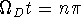, where n is an integer. This is incorrect, as it is missing a factor of 2 (which is implied by the discussion). The condition should instead read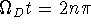, and the footnote should read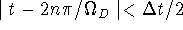. This error was first noted by James Behrens, and subsequently reported by several others.
• Chapter 5. On page 133, equation 5.25 should read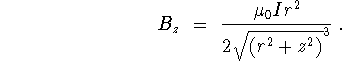Note that the correct result (the one given here) was used to generate the solid curve in Figure 5.16. Thanks to Bob Delaney for finding this and the next several errors.

• Chapter 7. On page 159, the line after equation 7.1 should read ``The arithmetic here is performed modulo m, with m typically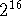or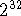...''
• Chapter 7. On page 171, on the fifth line the sentence should read ``Mixing will be roughly complete when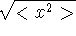is equal ...'' The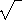is omitted in the book.
• Chapter 10. On page 285, on the line after equation 10.46, the sentence should read ``We can thus use (10.46) to obtain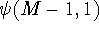, that is, the value of the new wave function one spatial unit in from the `right' boundary.''
• Appendix 4. On page 393, the second and third lines of the program listing should read

! x(i,n), y(i,n) = position of particle n
! i = 1 and 2 correspond to the old and current positions
• Appendix 1. This item concerns the Euler-Cromer and Verlet methods, and thus also is relevant to chapters 3 and 4, where the Euler-Cromer method is used. It turns out that the Euler-Cromer and Verlet methods are much more similar than is apparent (at least to me) at first sight. This can be seen as follows.

The Euler-Cromer method can be written as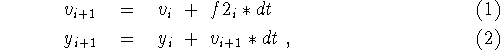where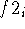is the second derivative of y at step i. This also implies that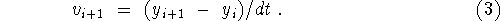The Verlet method can be written as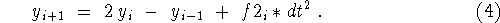If one eliminates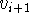from (2) one arrives at precisely (4). Hence, the two methods are equivalent with regards to y.

However, they are not necessarily the same with regards to the velocity. For the Verlet method it is best to obtain the velocity as a centered difference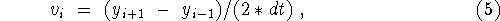which is different, and more accurate than, the Euler-Cromer expression.

Thanks to Sam Buss for pointing out the equivalence of the Euler-Cromer and Verlet methods.

• On page 138, 6th line from the bottom, ``appling'' should be ``applying.'' On p. 207, the 8th line from the top should read ``... and can be reviewed by reading ...'' On page 384, there is a typo in the comments for the waves-on-a-string program. 18 lines from the bottom it should read
! n = 1 = position two time steps ago

Thanks to Ramin Jamshidi for finding these errors.

Nick Giordano
Thu Aug 13 11:02:49 EST 1998